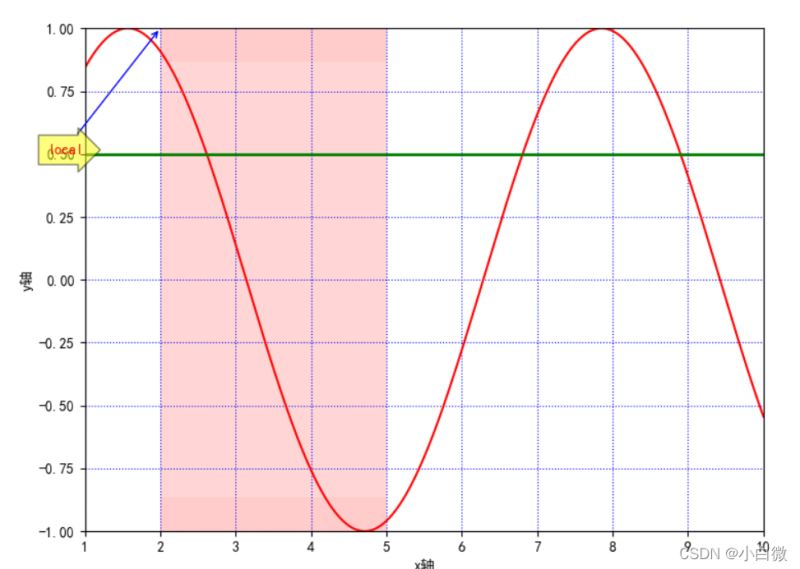﻿ Python数据可视化之matplotlib.pyplot绘图的基本参数详解_python_脚本之家
python# Python数据可视化之matplotlib.pyplot绘图的基本参数详解

matplotlib.pyplot模块是一个功能强大的画图模块,可以对画图的多个参数进行调整,下面这篇文章主要给大家介绍了关于Python数据可视化之matplotlib.pyplot绘图基本参数的相关资料,需要的朋友可以参考下

## 1.matplotlib简介

matplotlib 库是 Python 中绘制二维和三维图表的数据可视化工具

使用简单绘图语句实现复杂绘图效果

以交互式操作实现渐趋精细的图形效果

使用嵌入式 LaTex 输出具有印刷级别的图表、科学表达式和符号文本

对图表的组成元素实现精细化控制

• pyplot：面向当前图
• axes：面向对象
• Pylab：沿用 matlab 风格

```import matplotlib.pyplot as plt
import numpy as np```

## 2.图形组成元素的函数用法

plot()：展示变量的趋势变化

使用方法：plt.plot(x, y, c，ls, lw, label, alpha, **kwargs)

• x，y：x，y 轴上的数值
• c：设置颜色
• ls：折线图的线条风格
• lw：折线图的线条宽度
• label：标记图形内容的标签文本
• alpha：透明度
•  **kwargs：指定使用的是 line2D 属性

### 2.1. figure()：背景颜色

num ：

如果此参数没有提供，则一个新的 figure 对象将被创建，同时增加 figure 的计数数值，此数值被保存在 figure 对象的一个数字属性当中。如果有此参数，且存在对应 id 的 figure 对象，则激活对于 id 的 figure 对象。如果对应 id 的 figur 对象不存在，则创建它并返回它。如果 num 的值是字符串，则将窗口标题设置为此字符串

figsize：以英寸为单位的宽高，缺省值为 rc figure.figsize (1 英寸等于 2.54 厘米)

dpi：图形分辨率，缺省值为 rc figure.dpi

facecolor：背景色

```plt.figure(figsize=(10, 10))
x = np.linspace(0.05, 10, 1000)  # 在0.05到10的区间中，等差选取1000个，端点不属于
y = np.sin(x)
plt.rcParams['font.sans-serif'] = ['SimHei']
plt.rcParams['axes.unicode_minus'] = False
plt.plot(x, y,
color='red',
ls='-',
label='sinx')
plt.show()```### 2.2 xlim()和 ylim()：设置 x，y 轴的数值显示范围

使用方法：`plt.xlim(xmin,xmax)`

• xmin：x 轴上的最小值
• xmax：x 轴上的最大值

### 2.3 xlabel()和 ylabel()：设置 x，y 轴的标签文本

• fontsize：数字或者（small，large，medium）
• verticalalignment：距离坐标轴的位置（top，bottom，center，baseline）
• hoizontalalignment：位置（center，right，left）
• ratation：位置（vertical，horizontal，vertical）
• bbox：添加边框

### 2.5 axhline()：绘制平行于 x 轴额度水平参考线

y：水平参考线的出发点

```plt.figure(figsize=(10, 10))
x = np.linspace(0.05, 10, 1000)  # 在0.05到10的区间中，等差选取1000个，端点不属于
y = np.sin(x)
plt.rcParams['font.sans-serif'] = ['SimHei']
plt.rcParams['axes.unicode_minus'] = False
plt.plot(x, y,
color='red',
ls='-',
label='sinx')
plt.xlim(1, 10)
plt.ylim(-1, 1)
plt.xlabel('x轴')
plt.ylabel('y轴')
plt.grid(ls=':',
color='blue')  # 设置网格，颜色为蓝色
plt.axhline(0.5, color='green', lw=2, label="分割线")  # 绘制平行于x轴的水平参考线，绿色，名称
plt.show()```（上图中绿色的线即为axjline（）添加的参考线）

### 2.6 axvspan()：绘制垂直于 x 轴的参考区域

• xmin：参考区域的起始位置
• xmax：参考区域的终止位置
• facecolor：参考区域的填充颜色
• alpha：参考区域填充颜色的透明度,[0~1]

```plt.axvspan(xmin=2,
xmax=5,
facecolor='r',
alpha=0.2)  # 绘制垂直于x轴的参考区域```### 2.7 xticks(),yticks()

`plt.xticks(list(range(0, 12, 1)))  # 调整刻度范围和刻度标签`### 2.8 annotate()：添加图形内容细节的指向型注释文本

• s：注释文本内容
• xy：被注释的坐标点
• xytext：注释文字的坐标位置
• weight：设置字体线形(Ultralight，light，normal，regular，book，medium，roman，semibold，demibold，demi,bold，heavy，extrabold，black）
• color：设置字体颜色；也可以设置 RGB 或 RGBA 类型的颜色；但必须为[0,1]之间的浮点 数

xycoords= 参数如下

• figure points：图左下角的点
• figure pixels：图左下角的像素
• figure fraction：图的左下部分
• axes points：坐标轴左下的点
• axes pixels：坐标轴左下的像素
• data：使用被注释对象的坐标系统
• arrowprops：箭头参数，参数类型为字典 dict
• width：箭头的宽度
• shrink：总长度的一部分，从两端“收缩”
• facecolor：箭头颜色（如果设置了 arrowstyle 关键字，上面的参数都不可以用，可

• -
• ->
• -[
• |-|
• -|>
• <->
• <|-
• <|-|>
• fancy
• simple
• wedge）
```plt.annotate('local',
xy=(2, 1),
xytext=(0.5, 0.5),
weight='bold',
color='red',
xycoords="data",
arrowprops=
dict(arrowstyle="->", connectionstyle='arc3', color='b'),
bbox=
dict(boxstyle="rarrow",
fc="yellow",
ec='k',
lw=1,
alpha=0.5)
)```这里的黄色箭头和蓝色细长线即为参数方法添加的参数，实际使用过程中根据自己的实际所需使用，可以认为添加对图像的一些解释

### 2.9 bbox：给标题增加外框

（boxstyle：方框外形；circle：椭圆；darrow：双向箭头；larrow：箭头向左；rarrow：箭

### 2.10 . text()：添加图形内容细节的无指向型注释文本(水印）

x,y：表示坐标轴上的值

weight：

• ultralightlight
• normal
• regular
• book
• medium
• roman
• semibold
• demibold
• demi
• bold
• heavy
• extrabold
• black

xycoodrds：

• figure points：图左下角的点
• figure pixels：图左下角的像素
• figure fraction：图的左下部分
• axes points：坐标轴左下的点
• axes pixels：坐标轴左下的像素

data：使用被注释对象的坐标系统

arrowprops：箭头参数，参数类型为字典 dict

width：箭头的宽度

shrink：总长度的一部分，从两端“收缩”

facecolor：箭头颜色

bbox：给标题增加外框

boxstyle：方框外形

circle：椭圆

darrow：双向箭头

larrow：箭头向左

rarrow：箭头向右

round：圆角矩形

round4：椭长方形

roundtooth：波浪形边框 1

sawtooth：波浪形边框 2

square：长方形

```plt.text(1, 1,
"y=sinx",
weight='bold',
color ='b')```### 2.11. title()：添加图形内容的标题

`plt.title("正弦函数")`### 2.12. legend()：标示不同图形的文本标签图例

• best
• upper right
• upper left
• lower left
• lower right
• right
• center left
• center right
• lower center
• upper center
• center
`plt.legend(loc="lower left") # 设置图例位置`### 2.13 table()：向子图中添加表格

plt.table(cellText=None, cellColours=None, cellloc='right' ,colWidths=None,
rowLabels=None, rowColours=None, collLabels=None, colColours=None,
collloc='center', loc='bpttpm', bbox=None, edges='closed', **kwargs)

cellText：表格单元格文本。类型为二维字符串列表

cellColours：表格单元格背景色。类型为二位颜色值列表

cellloc：表格单元格文本的对齐方式。默认值为right

left

right

center

colWidths：表格单元格宽度。类型为浮点数列表

rowLabels：表格行表头文本。类型为字符串列表

rowColours：表格行表头背景色。类型为颜色列表

colLabels：表格列表头文本。类型为字符串列表

colLoc：表格行表头文本对齐方式。默认 right

left

right

center

colColours：表格列表头背景色。类型为颜色列表

loc：单元格相对于子图的位置

bbox：绘制表格的边界框，如果此参数不为 None，将会覆盖 loc 参数

edges：单元格边线，该属性会影响各类单元格背景色

BRTL

open

closed

horizontal

vertical

## 3. 完整代码显示

```import matplotlib.pyplot as plt
import numpy as np

plt.figure(figsize=(10, 10))
x = np.linspace(0.05, 10, 1000)  # 在0.05到10的区间中，等差选取1000个，端点不属于
y = np.sin(x)
plt.rcParams['font.sans-serif'] = ['SimHei']
plt.rcParams['axes.unicode_minus'] = False
plt.plot(x, y,
color='red',
ls='-',
label='sinx')
plt.xlim(1, 10)
plt.ylim(-1, 1)
plt.xlabel('x轴')
plt.ylabel('y轴')
plt.grid(ls=':',
color='blue')  # 设置网格，颜色为蓝色
plt.axhline(0.5, color='green', lw=2, label="分割线")  # 绘制平行于x轴的水平参考线，绿色，名称
plt.axvspan(xmin=2,
xmax=5,
facecolor='r',
alpha=0.2)  # 绘制垂直于x轴的参考区域
plt.axhspan(ymin=(-3**0.5)/2,
ymax=(3**0.5)/2,
facecolor='w',
alpha=0.2)

plt.legend(loc="lower left")  # 设置图例位置
plt.annotate('local',
xy=(2, 1),
xytext=(0.5, 0.5),
weight='bold',
color='red',
xycoords="data",
arrowprops=
dict(arrowstyle="->", connectionstyle='arc3', color='b'),
bbox=
dict(boxstyle="rarrow",
fc="yellow",
ec='k',
lw=1,
alpha=0.5)
)
plt.xticks(list(range(0, 12, 1)))  # 调整刻度范围和刻度标签
plt.text(1, 1,
"y=sinx",
weight='bold',
color ='b')
plt.title("正弦函数")
plt.show()```

```plt.rcParams['font.sans-serif'] = ['SimHei']
plt.rcParams['axes.unicode_minus'] = False```

-：实线样式
--：短横线样式
-.：点划线样式
：：虚线样式
.：点标记
O：圆标记
V：倒三角标记
^：正三角标记
<：左三角标记
>：右三角表示
1：下箭头标记13
2：上箭头标记
3：左箭头标记
4：右箭头标记
S：正方形标记
p：五边形标记
*：星形标记
H：六边形标记
+：加号标记
X：x 标记
D：菱形标记
|：竖直线标记
_：水平线标记

b 蓝色
g 绿色
r 红色
c 青色
m 品红色·
y 黄色
k 黑色
w 白色